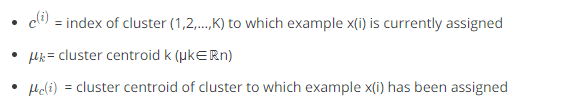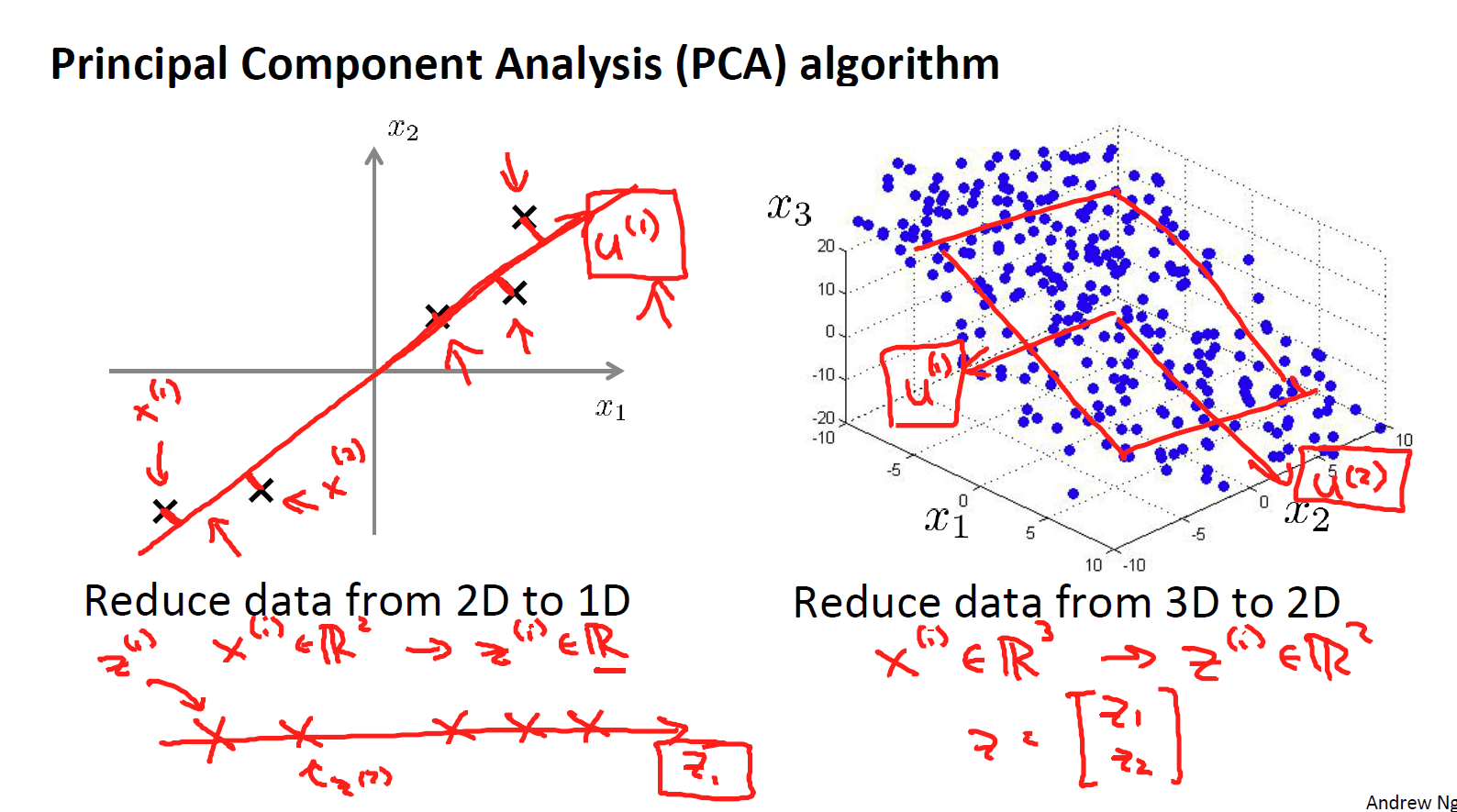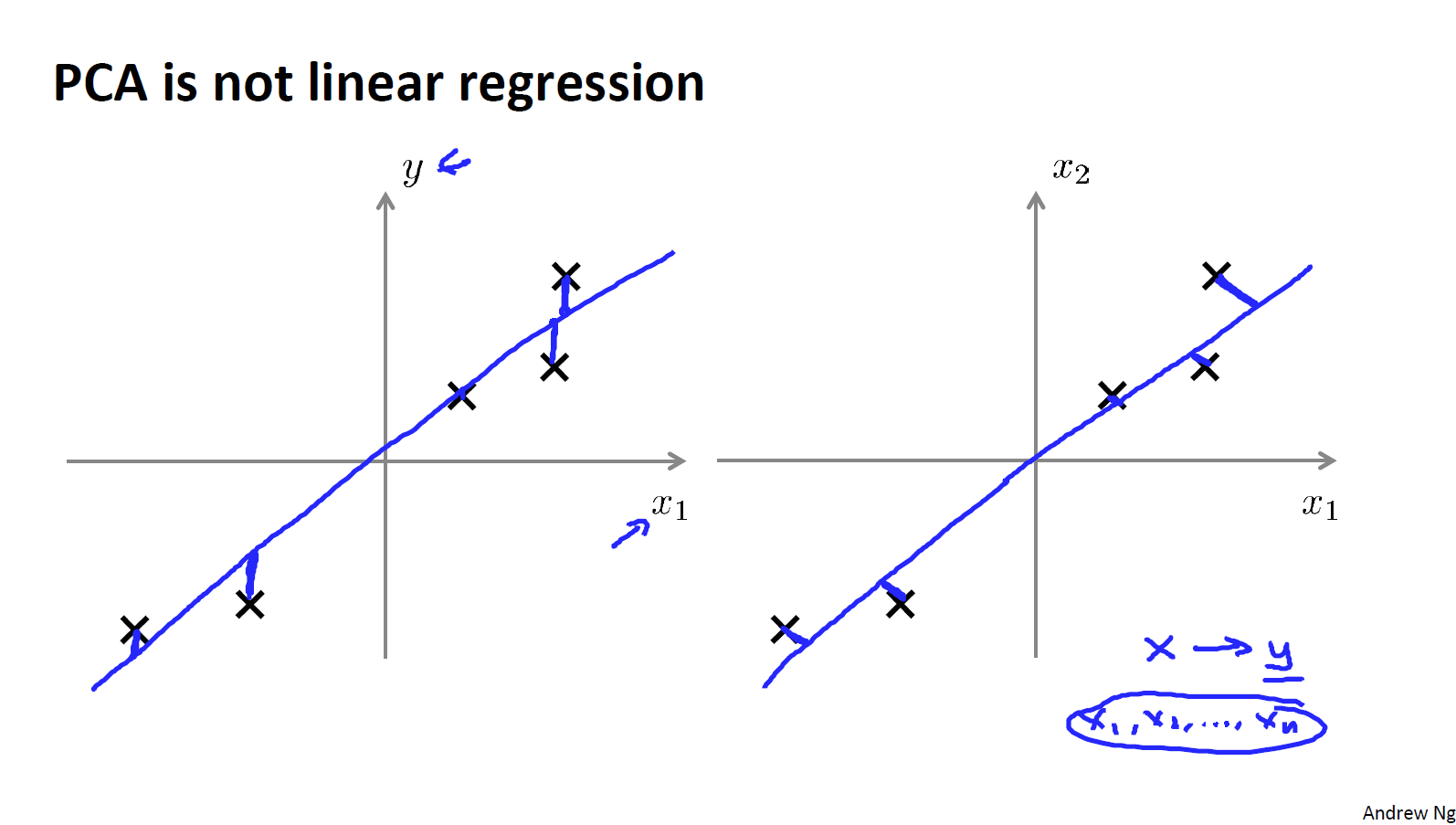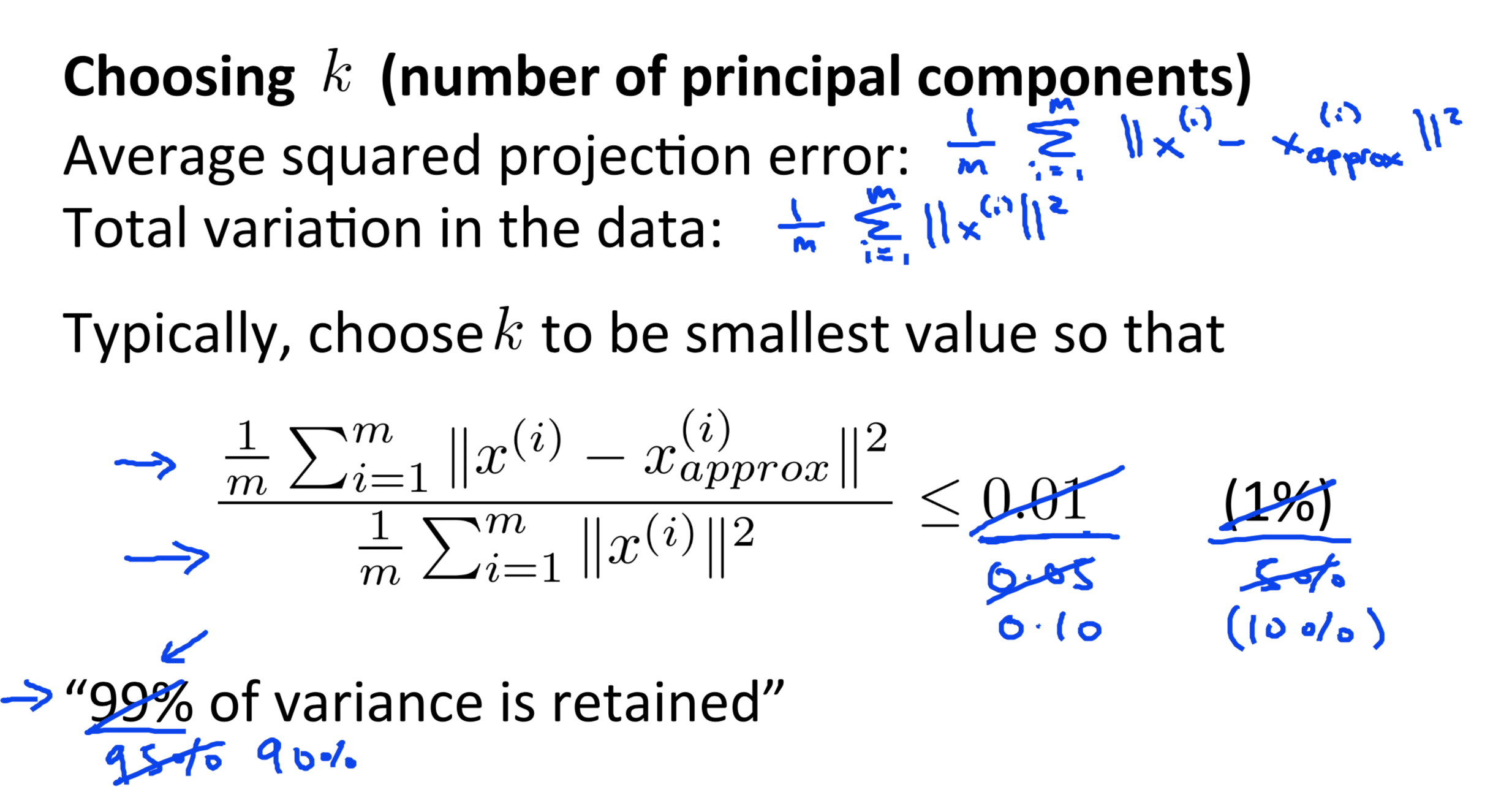# 吴恩达机器学习笔记-非监督学习

## 聚类

### K-means

K-Means算法是最流行和广泛使用的自动将数据分组到相干子集的算法，其算法的步骤主要是：

1. 首先选择 K 个随机的点，称为聚类中心（cluster centroids）；
2. 对于数据集中的每一个数据，按照距离K个中心点的距离，将其与距离最近的中心点关联起来，与同一个中心点关联的所有点聚成一类。
3. 计算每一个组的平均值，将该组所关联的中心点移动到平均值的位置。
4. 重复步骤2-4直至中心点不再变化。μ^1,μ^2,...,μ^k , 来表示聚类中心，用 c^{(1)},c^{(2)},...,c^{(m)} ,来存储与第 i 个实例数据最近的聚类中心的索引，K-均值算法的伪代码如下：

Randomly initialize K cluster centroids mu(1), mu(2), ..., mu(K)
Repeat:
for i = 1 to m:
c(i):= index (from 1 to K) of cluster centroid closest to x(i)
for k = 1 to K:
mu(k):= average (mean) of points assigned to cluster kJ(c^{(1)},...,c^{(m)},μ_1,...,μ_K)=\dfrac {1}{m}\sum^{m}_{i=1}\left| X^{\left( i\right) }-\mu_{c^{(i)}}\right| ^{2}

### 随机初始化

1. 我们应该选择 K<m，即聚类中心点的个数要小于所有训练集实例的数量
2. 随机选择 K 个训练实例。
3. 令 K 个聚类中心分别与这 K个训练实例相等

K-均值的一个问题在于它有可能会停留在一个局部最小值处，而这取决于初始化的情况。为了解决这个问题，我们通常需要多次运行K-均值算法，每一次都重新进行随机初始化，最后再比较多次运行K-均值的结果，选择代价函数最小的结果。这种方法在K较小的时候（2--10）还是可行的，但是K如果较大，这么做也可能不会有明显地改善。

for i = 1 to 100:
randomly initialize k-means
run k-means to get 'c' and 'm'
compute the cost function (distortion) J(c,m)
pick the clustering that gave us the lowest cost


### 选择聚类数

K的选择往往是任意的或者说是模糊不清的，通常是需要根据不同的问题人工进行选择的。选择的时候我们需要思考运用K-均值算法聚类的动机是什么，然后选择能最好服务于该目的标聚类数。## 降维

### 主成分分析问题1. 计算loss的方式不同（垂直）。
2. PCA没有标签Y（非监督）。### PCA算法

PCA 减少 n 维到 k 维：

\Sigma=\frac {1}{m}\sum^{n}_{i=1}\left( x^{(i)}\right) \left( x^{(i)}\right) ^{T}### 选择主成分的数量

1. 第一个是：PCA所做的是尽量最小化平均平方映射误差(Average Squared Projection Error)。
2. 第二个是：我还要定义一下数据的总变差(Total Variation)。它的意思是 “平均来看我的训练样本距离零向量多远？PCA算法主要有以下用途：

• 压缩：
• 减少内存和磁盘的占用
• 提升算法的速度
• 可视化：
• 降维到二维或者三维